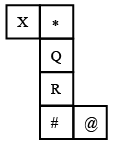Question 4

# Select the cube that can be formed by folding the given sheet (unfolded box) along the lines.Solution

When the given sheet is folded along the lines,

Q is opposite to #

R is opposite to $$*$$

X is opposite to @

From the first cube, Q is not opposite to #. So first cube cannot be formed.

From the second cube, R is not opposite to $$*$$. So second cube cannot be formed.

From the fourth cube, X is not opposite to @. So fourth cube cannot be formed.

$$\therefore\$$Third cube can be formed by folding the given sheet of paper.

Hence, the correct answer is Option C

• Free SSC Study Material - 18000 Questions
• 230+ SSC previous papers with solutions PDF